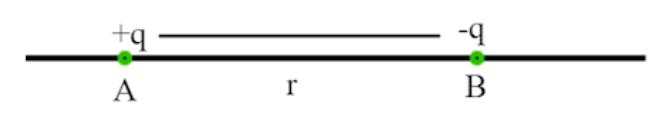QuestionAnswers

# The test charge used to measure electric field at a point should be____________A) Infinitely largeB) Vanishingly smallC) NegativeD) ZeroVerified
128.7k+ views
Hint: The concept of electric field is very important to study electrostatic phenomena. We know that electric force is developed between two charges when it is placed at a certain distance. This action-at-distance can be explained by using the concept of the electric field.

Complete step by step solution:
A test charge is a vanishingly small positive charge that is used to detect the presence of an electric field. The test charge should be as small as possible so that its presence does not affect the electric field due to the source charge.
The electric charge that produces the electric field is called a source charge.

$\therefore$ The test charge used to measure an electric field at a point should be vanishingly small. The correct answer is option (B).

The strength of the electric field is described by a quantity called electric field intensity or electric field strength. The electric field intensity at a point is defined as the force experienced by unit positive charge placed at that point.
If a small positive test charge +q are placed at a point P experiences a force F, then,
Then electric field intensity is given by,
$\overrightarrow E = \dfrac{{\overrightarrow F }}{q}$
Every charge Q creates an electric field in the space surrounding it. If another charge q is brought near Q then, the electric field of Q exerts a force on q.
Let us consider a point charge +Q placed at point O. Let P be the point at a distance r from O, where q is placed. Then
Electrostatic force between Q and q is given by,
$\overrightarrow F = \dfrac{1}{{4\pi {\varepsilon _0}}}\dfrac{{Qq}}{{{r^2}}}\widehat r$……………………. (1) Where $\widehat r$ is the unit vector directed from +Q to +q
Then electric field intensity is given by,
$\overrightarrow E = \dfrac{{\overrightarrow F }}{q}$
Now substitute equation (1) in above equation we get,
$\overrightarrow E = \dfrac{1}{{4\pi {\varepsilon _0}}}\dfrac{Q}{{{r^2}}}\widehat r$
Electric field due a point charge is given by,$\left| {\overrightarrow E } \right| = \dfrac{1}{{4\pi {\varepsilon _0}}}\dfrac{Q}{{{r^2}}}$

Note:
The electric field intensity at a point is defined as the force experienced by unit positive charge placed at that point.
The electric field due to a charge is the space around the charge in which any other charge experiences a force of attraction or repulsion.